# Number Chart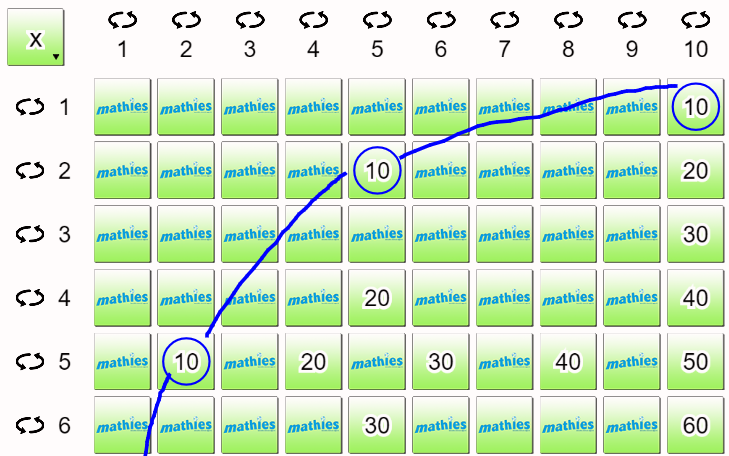Click to Open in a new browser window (Desktop or Mobile)Click to Open in French in a new browser windowClick to view options for offline use (more details)View entry on mathies.ca

Version française de cette page

Description
Examples
Features

## Number Chart

The mathies interactive Number Chart tool includes two number charts (0-99 and 1-100), an addition chart (to 12 + 12), and a multiplication chart (to 12 x 12). Flip individual cards, rows, columns or all cards at once.

### Number Chart Considerations

• Zero is not a counting number. So, the 1-100 chart might be preferred for counting activities.
• Zero is a number, in its own right, not just a digit in values such as 50. Seeing 0 as the first value in the 0-99 chart helps to emphasize this fact.
• On a 0-99 chart, all the numbers in each decade appear in the same row (e.g., 20, 21, 22, ...29). Values in any given row all contain the same number of groups of ten and then some number of ones. Seeing all the values from the same decade in the same row helps reinforce place value concepts.
• In both charts, moving one square to the right is equivalent to adding 1 while moving one square to the left is equivalent to subtracting 1.
• In both charts, moving one row directly down from any given value is the same as adding 10 while moving one row above a given value is the same as subtracting 10.
• Questions such as 16 - 16 can be solved using the 0-99 chart but not the 1-100 chart.
• Questions such as 86 + 14 can be solved using the 1-100 chart but not the 0-99 chart.
• See Link #3 for more details.

### Mathematical Concepts:

Number charts offer versatile ways to explore numerous math skills such as:
• counting
• patterning
• developing and understanding addition strategies
• developing and understanding multiplication strategies
• problem solving

Addition and multiplication charts provide opportunities for immediate feedback as students practise addition and multiplication facts. These charts can also be used to support students in developing and understanding various strategies for adding and multiplying.

Students’ learning can be scaffolded in different ways providing support for diverse learning needs (e.g., hide rows and columns to consider fewer cards).

top

### Connecting Fundamental Math Concepts with the mathies Number Chart

Fundamental Concepts and Skills (Link #6) Number Chart Connections
Working with numbers:
Understanding and using numbers (e.g., being able to read, represent, count, order, estimate, compare, compose, decompose, and recompose numbers).
Number charts can be used to:
• support students in numeral recognition
• practise counting and skip counting
• compare numbers by considering their relative position in the chart
Recognizing and applying understanding of number properties:
Understanding how numbers behave in operations and drawing on that understanding to master math facts and perform calculations.
Number and Operation charts can be used to:
Mastering math facts:
Understanding and recalling math facts, using a variety of strategies.
Operation charts are good tools to use to practise addition and multiplication facts.
Developing mental math skills:
Doing calculations in the mind, with little or no use of paper and pencil or calculator.
Using visual tools when learning to perform mathematical operations allows students to draw on these mental models and visualizations to perform mental calculations.
The Number Chart can be used to practise a variety of strategies including:
• decomposing numbers into friendly numbers to make calculations easier
• compensating by adding then subtracting the same amount
Developing proficiency with operations:
Performing calculations with ease, precision, and consistency and with a general understanding of number and operations, number properties, and their appropriate application in problem solving.
Students might use the operations charts to recognize the inverse relationship between addition and subtraction (e.g., since 4 + 5 = 9, then 9 - 5 = 4) as well as the inverse relationship between multiplication and division (e.g., since 7 x 8 = 56, then 56 ÷ 7 = 8).
top

## Examples

### Counting and Numeral Recognition

Number charts can be used to support students in developing counting and numeral recognition skills.

Activities Using the Number Chart from 1-100
• Ask students to determine missing numbers on the chart.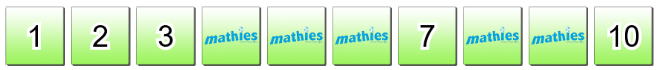• Asking students to work with more rows in the chart changes the level of difficulty.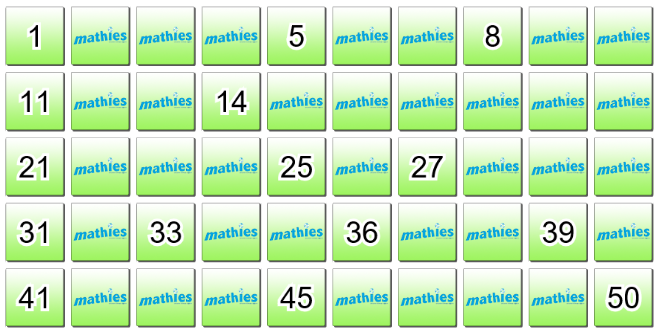• Have students mark each number (here the circle stamp is used) as they skip count by various values.
Encourage them to look for patterns.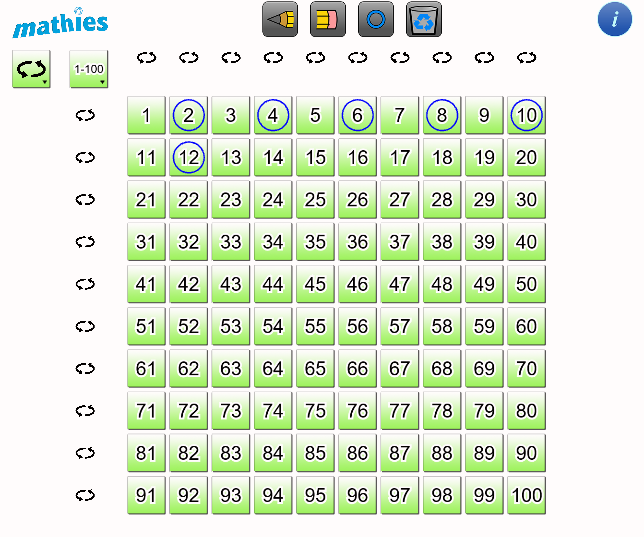• Eventually, encourage students to start skip counting at numbers other than the typical 1, 2, 5, or 10 so they can see the patterns shift. This example shows starting at three and then skip counting by five.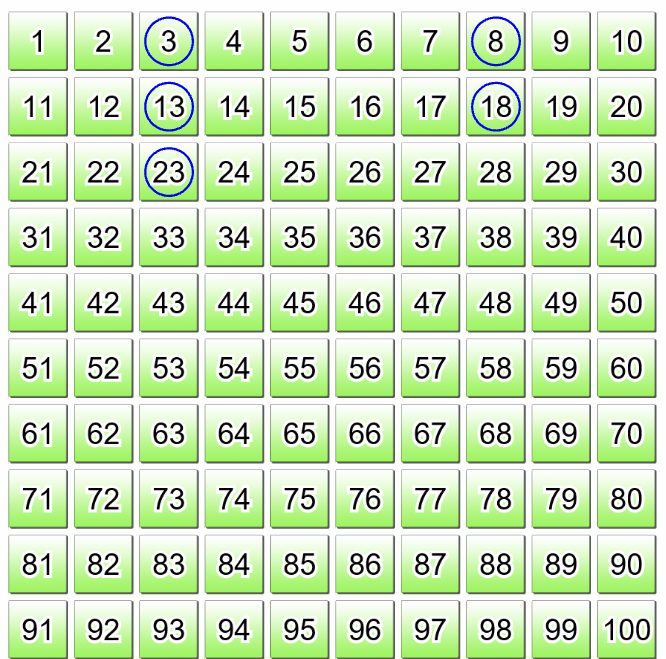top

### Patterning

Number charts can be used to create a range of patterns from simple AB patterns to growing or shrinking patterns as well as to really delve into multiples. Students can use the charts to help identify, extend, and create patterns.

Patterns Using the 0-99 Number Chart
• Flip all the numbers with a 3 as the ones or tens digit and look for a pattern. Repeat with 7 and compare. Try other numbers. What happens? Why?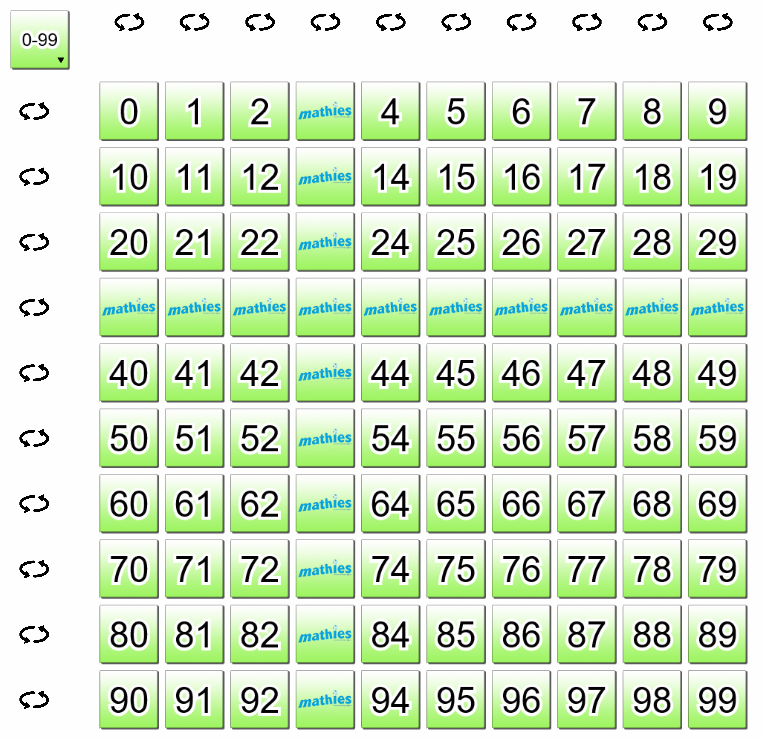• Play with challenges such as cover all numbers with:
• digits that add to 9, or
• a tens digit that is larger than the ones digit, or
• a tens digit that is smaller than the ones digit.

Patterns Using Either Number Chart
• Hide all the cards. Flip two cards, skip one, flip two, skip one. Ask students to extend this repeating pattern. Can they predict what values will be on the hidden cards before flipping them? Once the pattern has been extended for the entire chart, ask students to think about the values on the cards that remain hidden. Students can then use the Flip All option to flip all the cards in the chart. What pattern do they notice in the cards that are now showing? Have them try a different repeating pattern of their own and look for patterns.
• Give students pattern challenges to mark on their boards. Have them determine the pattern rule and the next number(s) in the pattern.
e.g., 19, 16, 13, 10… or 21, 28, 35, 42…
• Consider the diagonals in the 0-99 chart. What patterns do you notice? Consider the diagonals in the 1-100 chart. What patterns do you notice? How are the patterns in the two charts the same? Different? (Adapted from Link #3)

top

Activities Using Either Number Chart
• Consider patterns that involve moving a row and one or two squares (i.e., adding or subtracting 11 or 12). Help the students link this to their mental math skills.

• Compute sums such as 23 + 36 by decomposing addends into friendly numbers.
Add 23 + 30 by moving down three rows of ten then add on 6 more by moving to the right six cards.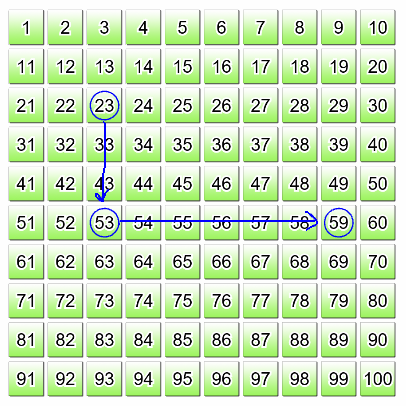• Compute sums such as 38 + 8 by compensating (adding and then subtracting the same amount).
e.g.,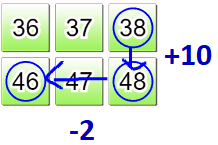• Think of 38 + 8
• as 38 + (8 + 2) - 2
• or 38 + 10 - 2.

• Demonstrate the associative property of addition.
e.g., Determine (7 + 6) + 4 and show the result is the same as 7 + (6 + 4).

• Explicitly discuss various addition strategies.(Source: Link #4) Indicate the addition facts where these strategies apply on the addition chart. Some addition facts can be determined using more than one strategy. Look for visual patterns for each strategy.
• One-more-than and two-more-than facts (e.g., 1 + 5 and 7 + 2)
• Doubles (e.g., 2 + 2 and 8 + 8)
• Near doubles (e.g., 6 + 7 and 5 + 4)
This strategy involves sums where one addend is one more than the other.
The strategy is to double the smaller number and add one more or to double the larger number and take away one.
• Anchor-of-Ten Facts (e.g., 1 + 9 and 8 + 2)
• Make-Ten Facts (e.g., 3 + 8 and 9 + 5)
This strategy involves adding onto either 8 or 9 to make 10, then adding on the rest.
e.g., For 9 + 5, start with 9 and add on 1 to make 10, then add on 4 more to get 14.

• Demonstrate the commutative property of addition.
In this example, the following pairs of questions are marked: 3 + 4 and 4 + 3, 1 + 10 and 10 + 1, 9 + 6 and 6 + 9.
Mark several more pairs of questions on the chart. What pattern do you notice?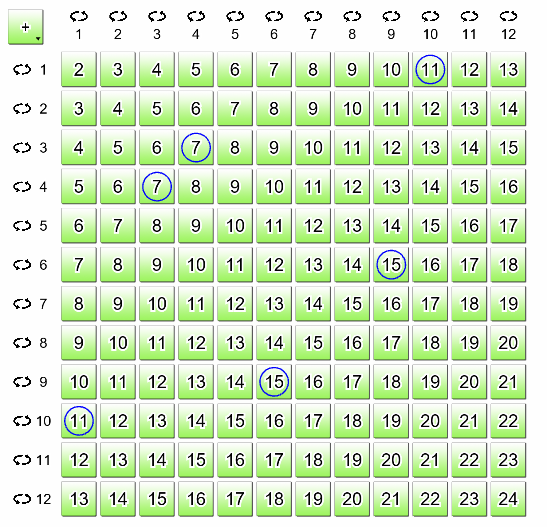• How many different ways can you find two numbers that add to 12? Mark each sum on the addition chart. Repeat using a different sum. What patterns do you notice?

Start by hiding all the cards in the chart. Choose an addition fact. Determine the sum. Flip the corresponding card to check.
top

### Multiplication

Using one of the hundreds charts, assign a number and have the students find and flip that number and its multiples.

Activities Using the Multiplication Chart
• Look for patterns connected to various factors. Start with the multiples of 2, 5, and 10 and gradually add the other factors. Describe the pattern in the chart for each factor.

• Start by hiding all the numbers in the chart. Focus on one factor by considering one row or one column at a time. Expose some of the numbers (products) and determine each of the missing products. Flip the card to check. Think about the strategies used. Discuss.
e.g., Consider products where one factor is 6. The products 6, 12, 30 and 60 are showing. Determine the remaining multiples of 6.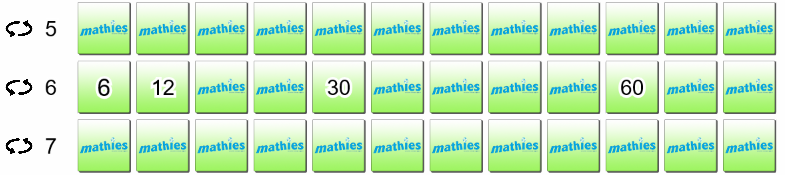• Start by hiding all the numbers in the chart. Randomly select a digit from 0 to 9. Flip over all product cards that contain a digit in the product that matches the randomly selected digit. Discuss: How do you know you found all the products containing the indicated digit? What strategies did you use? (Source: Link #1)

• Demonstrate the commutative property of multiplication.
Mark pairs of questions such as: 3 x 4 and 4 x 3, 1 x 10 and 10 x 1, 9 x 6 and 6 x 9.
Mark several more pairs of questions on the chart. What pattern do you notice?

• Demonstrate the associative property of multiplication.
e.g., Determine (3 x 2) x 5 and show the result is the same as 3 x (2 x 5).

• Practise multiplication facts.
Start by hiding all the cards in the chart. Choose a multiplication fact. Determine the product. Flip the corresponding card to check.
top

### Problem Solving

Using a Number Chart
•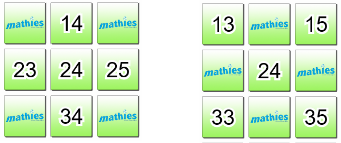A Cross pattern is a square plus the four squares directly up, down, left, and right from it.
An X pattern is a square plus the four touching it diagonally.
Choose any square that is not on an edge of the hundred board (use either hundreds chart). Find its Cross and X patterns and add up their sums. Can you explain why they add up to the same number? Can you find any other patterns that work that way? Can you figure out how to predict the Cross or X pattern sum for any number? (Source: Link #2)

• Start with all the cards on a 1-100 chart face up. Flip all the multiples of 2, except for 2 itself. Move to the next number that is still face up. Flip all the multiples of this value excluding the value itself. Continue until all possible cards have been flipped. Consider the remaining face up cards. What property do they share? Does the number 1 belong?

• Play "Guess the Number"
• e.g., The number is greater than 5 x 7.
• The number is less than 120 - 30.
• The number is not a multiple of 10.
• The sum of the numbers' digits is 11.
• I say this number when I skip count by 5.
• What is the number?

• Create your own "Guess the Number" puzzle. (Based on Link #5)

• Use the addition chart to show that the sum of two odd numbers is always even. What about the sum of two even numbers? The sum of an even and an odd number? Convince a friend that these generalizations are true.
top

## Features

Buttons Description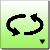### Flip Options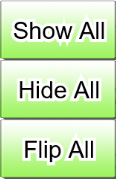Choose between these options:
• show the values on all the cards in the chart
• hide the values on all the cards in the chart
• flip all the cards in the chart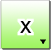### Chart Type Options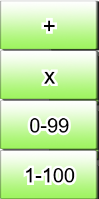Choose between these options:
• addition facts to 12 + 12
• multiplication facts to 12 x 12
• hundreds chart showing 0-99
• hundreds chart showing 1-100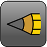### Draw Mode

Draw freehand by clicking and dragging.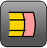### Erase Mode

Erase freehand drawings and circles by dragging the eraser around the number chart.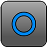### Circle Stamp

Either show or hide a circle on cards by:
• clicking individual cards
• clicking and dragging over multiple cards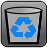### Recycle

Remove all annotation (i.e., freehand drawing and circle stamps).### English/French

Switch between English and French text.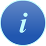### Information

Open a dialog with a link to this support page, a feedback form as well as copyright details and version number.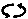### Flip Button

Click to flip all the cards in the corresponding row or column.
top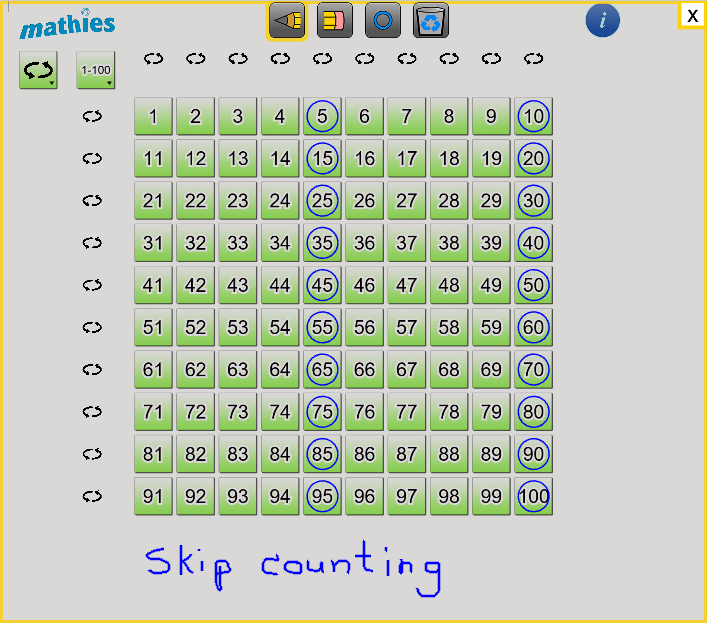### Annotation Mode

Click the pencil, eraser or circle button to enable annotation mode.
A grey mask with a yellow border appears to indicate the tool is in annotation mode.
To exit annotation mode and resume interacting with the number chart, click the x in the top right corner of the mask. Alternatively, click again on the highlighted button to exit annotation mode.

### URL Parameters

URL parameters allow for the tool to be opened with certain features preset.
e.g., Click on the following link:
https://mathies.ca/tools/NumberChart/index.html?title=Number%20Chart&language=fr&show=false&chart=100
The Number Chart tool will open showing the French version of the 1-100 chart with all the cards face down.
Notice that the parameter section of the URL starts with a ? and can include any of the four optional parameters in any order. The symbol & must separate parameters.
 Parameter Description Allowed Values title Sets the text that appears in the browser tab title.Default: Number%20Chart, where %20 indicates a space Simple Text language Determines the language setting for the tool.Default: en (English) en fr show Determines whether or not the tool opens with all the cards showing face up.Default: true true false chart Determines the type of chart.Default: mul (multiplication chart) add mul 99 100
top

To be among the first to find out about latest digital tool developments, sign up for our email list at https://mathclips.ca/WhatsNewEmailList.html.
Follow @ONmathies on Twitter and use #ONmathies to share your work or see what others are doing. Leave feedback.